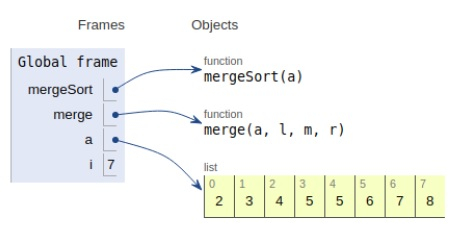# Python Program for Iterative Merge Sort

Problem statement − We are given an array, we need to sort it using the concept of merge sort by iteration.

Here we place the maximum element at the end. This is repeated until the array is sorted.

Now let’s observe the solution in the implementation below −

## Example

# iterative way
def mergeSort(a):
current_size = 1
# traversing subarrays
while current_size < len(a) - 1:
left = 0
# subarray being sorted
while left < len(a)-1:
# calculating mid value
mid = left + current_size - 1
# current_size
right = ((2 * current_size + left - 1, len(a) - 1)[2 * current_size + left - 1 > len(a)-1])
# Merge
merge(a, left, mid, right)
left = left + current_size*2
# Increasing sub array size
current_size = 2 * current_size
# Merge
def merge(a, l, m, r):
n1 = m - l + 1
n2 = r - m
L =  * n1
R =  * n2
for i in range(0, n1):
L[i] = a[l + i]
for i in range(0, n2):
R[i] = a[m + i + 1] i, j, k = 0, 0, l
while i < n1 and j < n2:
if L[i] > R[j]:
a[k] = R[j]
j += 1
else:
a[k] = L[i]
i += 1
k += 1
while i < n1:
a[k] = L[i]
i += 1
k += 1
while j < n2:
a[k] = R[j]
j += 1
k += 1
# Driver code
a = [2,5,3,8,6,5,4,7]
mergeSort(a)
print("Sorted array is:")
for i in range(len(a)):
print (a[i],end=" ")

## Output

Sorted array is
2 3 4 5 5 6 7 8All the variables are declared in the local scope and their references are seen in the figure above.

## Conclusion

In this article, we have learned about how we can make a Python Program for Iterative Merge Sort